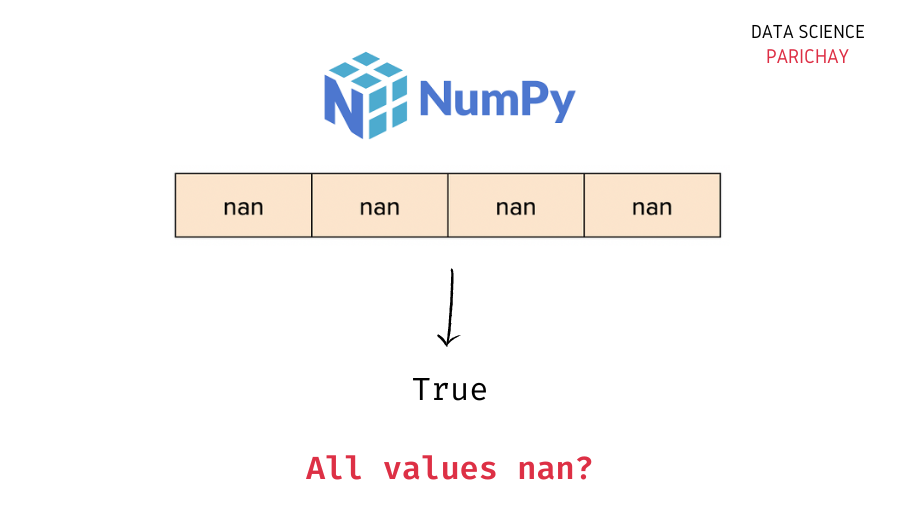# Numpy – Check if Array is all NaN

To check if all the values in a Numpy array are NaN or not, you can use a combination of the `numpy.isnan()` function and the `all()` function. The idea is to check if each value in the array is nan or not using `numpy.isnan()` which results in a boolean array and check if all the values in the resulting boolean array are `True` or not using the `all` function.

The following is the syntax –

```import numpy as np

# check if numpy array is all zero
np.isnan(ar).all()```

Alternatively, you can iterate through the array and return `False` if you encounter any non-NaN value.

Let’s now look at the methods mentioned above with the help of some examples.

## Example 1 – Check if the Array is all NaN using `all()` function

If you compare an array with a scaler value, the resulting array would be a boolean array with `True` for the array values that were equal to the scaler value and `False` otherwise.

In this method, we compare the array with `np.nan` and check if all the values in the resulting boolean array are `True` or not using the numpy array `all()` function.

Let’s look at an example

```import numpy as np

# create two arrays
ar1 = np.array([np.nan, np.nan, np.nan, np.nan])
ar2 = np.array([np.nan, 0, 1, np.nan])

# check if array is all NaN
print(np.isnan(ar1).all())
print(np.isnan(ar2).all())```

Output:

📚 Data Science Programs By Skill Level

Introductory

Intermediate ⭐⭐⭐

🔎 Find Data Science Programs 👨‍💻 111,889 already enrolled

Disclaimer: Data Science Parichay is reader supported. When you purchase a course through a link on this site, we may earn a small commission at no additional cost to you. Earned commissions help support this website and its team of writers.

```True
False```

Here, we created two arrays. `ar1` with all values as nan and `ar2` with only some values as nan (not all) and then checked if these arrays are all nan or not using our method.

We get `True` for `ar1` and `False` for `ar2`, which is the correct result.

## Example 2 – Iterate through the array

Alternatively, we can iterate through the entire array, element by element, and check if each element is nan or not using the `numpy.isnan()` function. If we encounter a non-nan element, we return `False`.

```import numpy as np

# create two arrays
ar1 = np.array([np.nan, np.nan, np.nan, np.nan])
ar2 = np.array([np.nan, 0, 1, np.nan])

# function to check if array is all nan
def is_array_all_nan(ar):
if len(ar) == 0:
return False
for val in ar:
if np.isnan(val):
continue
else:
return False
return True

# check if array is all zero
print(is_array_all_nan(ar1))
print(is_array_all_nan(ar2))```

Output:

```True
False```

We get the same result as above. You can think of this method as a more verbose version (and less optimized) version of method 1.

You might also be interested in –

•